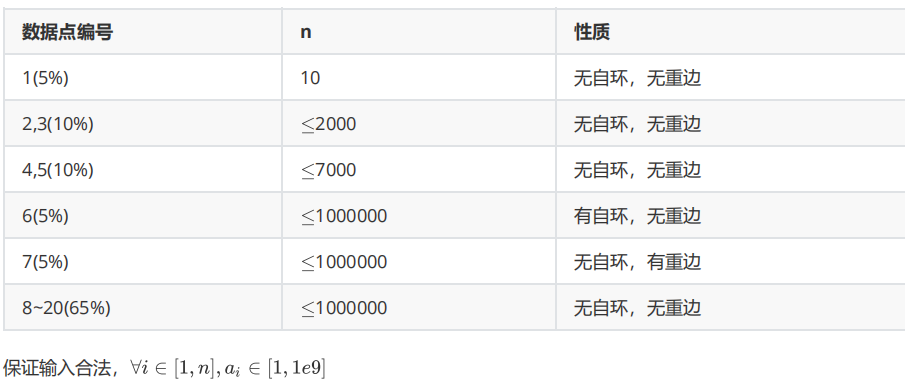## jzoj 5905.【NOIP2018模拟10.15】黑暗之魂(darksoul) 基环树+_Amber_lylovely的博客-程序员宅基地

Description

oi_juruo热爱一款名叫黑暗之魂的游戏。在这个游戏中玩家要操纵一名有 点生命值的无火的余灰在一张地图中探险。地图中有n个篝火（也就是存档点）。在篝火处休息可以将生命值恢复满。每个篝火都会向其他篝火的其中之一连有一条通道（显然，通道是双向的），这些篝火之间都相互可达。也就是说，这是一张n个点，n条边的无向连通图。每条通道里都有一些怪物，经过oi_juruo的分析，他得到了每条边的怪物会对他造成的伤害值 .为了向oier们表演他高超的游戏技巧，他要从任意一个篝火跑到任意另一个篝火而不在之间的篝火休息，在此期间，他会和他经过的通道中的怪物战斗并损失 的生命值。现在oi_juruo想知道，他的生命值 至少为多少，才能完成任意一条旅途。oi_juruo并不傻，他会走最安全的路。本题时限为3000ms

Input

Output

Sample Input

5
1 2 2
2 3 2
3 4 2
1 4 1
4 5 4

Sample Output

8

Data Constraint#include <iostream>
#include <cstdio>
#include <cmath>
#include <vector>
#include <algorithm>
#define LL long long

const int maxn=1e6+7;

using namespace std;

int ls[maxn],dfn[maxn],low[maxn],belong[maxn];
bool vis[maxn];
int id[maxn],q[maxn*2];
LL ans,f[maxn*2],size[maxn*2],s[maxn*2],dis[maxn*2],sumdis;

struct edge{
int y,w,next;
}g[maxn*2];

struct node{
int x,y,w;
}a[maxn];

vector <int> sta;

{
int x=0,f=1;char ch=getchar();
while (ch<'0'||ch>'9'){if(ch=='-')f=-1;ch=getchar();}
while (ch>='0'&&ch<='9'){x=x*10+ch-'0';ch=getchar();}
return x*f;
}

{
g[++cnt]=(edge){y,w,ls[x]};
ls[x]=cnt;
}

void tarjan(int x,int fa)
{
dfn[x]=low[x]=++cnt;
vis[x]=1; sta.push_back(x);
for (int i=ls[x];i>0;i=g[i].next)
{
int y=g[i].y;
if (y==fa) continue;
if (!dfn[y])
{
tarjan(y,x);
low[x]=min(low[x],low[y]);
}
else
{
if (vis[y]) low[x]=min(low[x],dfn[y]);
}
}
if (low[x]==dfn[x])
{
bcnt++;
int y=0,flag=0;
if (sta.back()!=x) flag=1,cir=bcnt;
while (y!=x)
{
y=sta.back();
sta.pop_back();
belong[y]=bcnt;
vis[y]=0;
if (flag) id[++tot]=y;
}
}
}

void dfs(int x,int fa)
{
LL max1=0,max2=0;
for (int i=ls[x];i>0;i=g[i].next)
{
int y=g[i].y;
if ((belong[y]==cir) || (y==fa)) continue;
dfs(y,x);
LL d=f[y]+g[i].w;
if (d>max1)
{
max2=max1;
max1=d;
}
else if (d>max2) max2=d;
}
ans=max(ans,max1+max2);
f[x]=max1;
}

bool cmp(node a,node b)
{
if (a.x==b.x)
{
if (a.y==b.y) return a.w<b.w;
return a.y<b.y;
}
return a.x<b.x;
}

int main()
{
freopen("darksoul.in","r",stdin);
freopen("darksoul.out","w",stdout);
for (int i=1;i<=n;i++)
{
if (a[i].x>a[i].y) swap(a[i].x,a[i].y);
}
sort(a+1,a+n+1,cmp);
for (int i=1;i<=n;i++)
{
if (a[i].x==a[i].y) flag=1;
else
{
if ((a[i].x==a[i-1].x) && (a[i].y==a[i-1].y)) flag=1;
else
{
}
}
}
if (flag)
{
cir=1;
dfs(1,0);
printf("%lld",ans+1);
return 0;
}
tarjan(1,0);
for (int i=1;i<=tot;i++) dfs(id[i],0),size[i]=f[id[i]];
for (int i=1;i<=tot;i++)
{
int d;
if (i==1) d=id[tot];
else d=id[i-1];
for (int j=ls[id[i]];j>0;j=g[j].next)
{
if (g[j].y==d)
{
dis[i]=(LL)g[j].w;
break;
}
}
}
for (int i=tot+1;i<=2*tot;i++) dis[i]=dis[i-tot],size[i]=size[i-tot];
for (int i=1;i<=2*tot;i++) s[i]=s[i-1]+dis[i];
sumdis=s[tot];
for (int i=2;i<=2*tot;i++)
{
q[++tail]=i;
}
printf("%lld",ans+1);
}


### Node.JS+Vue+Vite+D3可视化_追风筝的人i的博客-程序员宅基地_node vite

Node.JS+Vue+Vite+D3可视化——mac从 Vite 与 Vue 开始的 D3 数据可视化之旅（MAC）准备些啥呢？前期工作Node.jsyarngitVite站Vue+D3可视化安装d3依赖安装axios从 Vite 与 Vue 开始的 D3 数据可视化之旅（MAC）本文章用于入门学习Vue和Vite的搭建，同时使用D3实现数据可视化。准备些啥呢？首先准备好我们旅行的行李清单Node.js ，因为mac自带，所以我就直接使用的自带；npm or yarn:包管理工具，这里我使用

### 凯恩帝绝对坐标清零_凯恩帝 K90T i 数控车床系统使用手册分享_Oliverzzzhang的博客-程序员宅基地

A)机床实际运动方法1、可调整进给倍率2、采用单程序段，即是每按一次启动键后刀具走一个动作(执行一个程序段)后停止，再按启动键后刀具走下一个动作后(执行下一个程序段)停止，这样可以检查程序。B)机床不动， 观察显示位置变化或通过图形功能， 观察加工时的刀具轨道的变化。1.5. 数据的显示和设定：(1) 刀具补偿的显示和设定方法。(2) 参数的显示和设定。(3) 用诊断参数判断机床的输入输出口信号状...

### du和df_我什么都布吉岛的博客-程序员宅基地

//获得二维码 public function qrcode() { header('content-type:text/html;charset=utf-8'); //配置APPID、APPSECRET $id=$_GET['uid']; \$APPID = C('WX_APPID');...